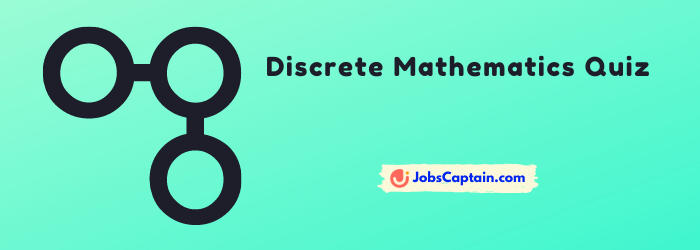# Discrete Mathematics Questions and AnswersYou are welcome to this quick Discrete Mathematics GK Quiz. As we know Maths quizzes are very important for all class students and also for competitive exams.

These are the selective and important questions of Discrete Mathematics. Let’s get started.

Question 1: Set of positive integers is known as?

(A) Finite

(B) Infinite

(C) Subset

(D) None

(B) Infinite

Question 2: Which is the complement of set A?

(A) A – B

(B) B – A

(C) A – U

(D) U – A

(D) U – A

Question 3: If a set contains 3 elements then the number of subsets is?

(A) 5

(B) 9

(C) 8

(D) 12

(C) 8

Question 4: How many bytes are required to encode 2000 bits of data?

(A) 2

(B) 4

(C) 6

(D) 8

(A) 2

Question 5: Floor (2.4) + Ceil (2.9) is equal to

(A) 9

(B) 3

(C) 5

(D) 7

(C) 5

Question 6: Which is an error-correcting code?

(A) Gray code

(B) Error deducting code

(C) Hamming code

(D) None

(C) Hamming code

Question 7: The sum of squares of the first n natural numbers is given by?

(A) n(n-1)/2(2n+1)

(B) n(n+1)(2n+1)/6

(C) n2(n+1)(2n+1)/6

(D) None

(B) n(n+1)(2n+1)/6

Question 8: A matrix having many rows and one column is known as?

(A) Column matrix

(B) Row matrix

(C) Row-Column matrix

(D) Diagonal matrix

(A) Column matrix

Question 9: If the determinant of a matrix A is Zero then?

(A) A is a non-Singular matrix

(B) A is a Singular matrix

(C)  Row matrix

(D) Column matrix

(B) A is a Singular matrix

Question 10: A continuous non – intersecting curve in the plane whose origin and terminus coincide?

(A) Planar

(B) Tree

(C) Euler

(D) Jordan

(D) Jordan

Question 11: The number of leaf nodes in a complete binary tree of depth d is?

(A) 2d+1

(B) 2d

(C) 2(d+1)

(D) d+2

(B) 2d

Question 12: In how many ways can a president and vice president be chosen from a set of 30 candidates?

(A) 870

(B) 880

(C) 890

(D) 878

(A) 870

Question 13: The number of colours required to properly colour the vertices of every planar graph is?

(A) 2

(B) 4

(C) 5

(D) 6

(C) 5

Question 14: In an undirected graph the number of nodes with an odd degrees must be?

(A) Zero

(B) Odd

(C) Even

(D) Prime

(C) Even

Question 15: The power set of the empty sets has exactly _____ subset.

(A) 2

(B) 3

(C) 4

(D) 5

(A) 2

Question 16: Discrete Mathematics is a branch of mathematics involving discrete elements that use?

(A) Algebra

(B) Arithmetic

(C) Both A and B

(D) None

(C) Both A and B

Question 17: In how many categories, Mathematics can be broadly classified into?

(A) 4

(B) 5

(C) 3

(D) 2

(D) 2

Question 18: How many bits string of length 4 are possible such that they contain 2 ones and 2 zeroes?

(A) 6

(B) 7

(C) 8

(D) 9

(A) 6

Question 19: Which of the following bits is the negation of the bits “010110”?

(A) 111111

(B) 101001

(C) 101101

(D) 111001

(B) 101001

Question 20: Which of the following involves distinct values i.e. between any two points?

(A) Continuous Mathematics

(B) Non-Continuous Mathematics

(C) Discrete Mathematics

(D) Non-Discrete Mathematics